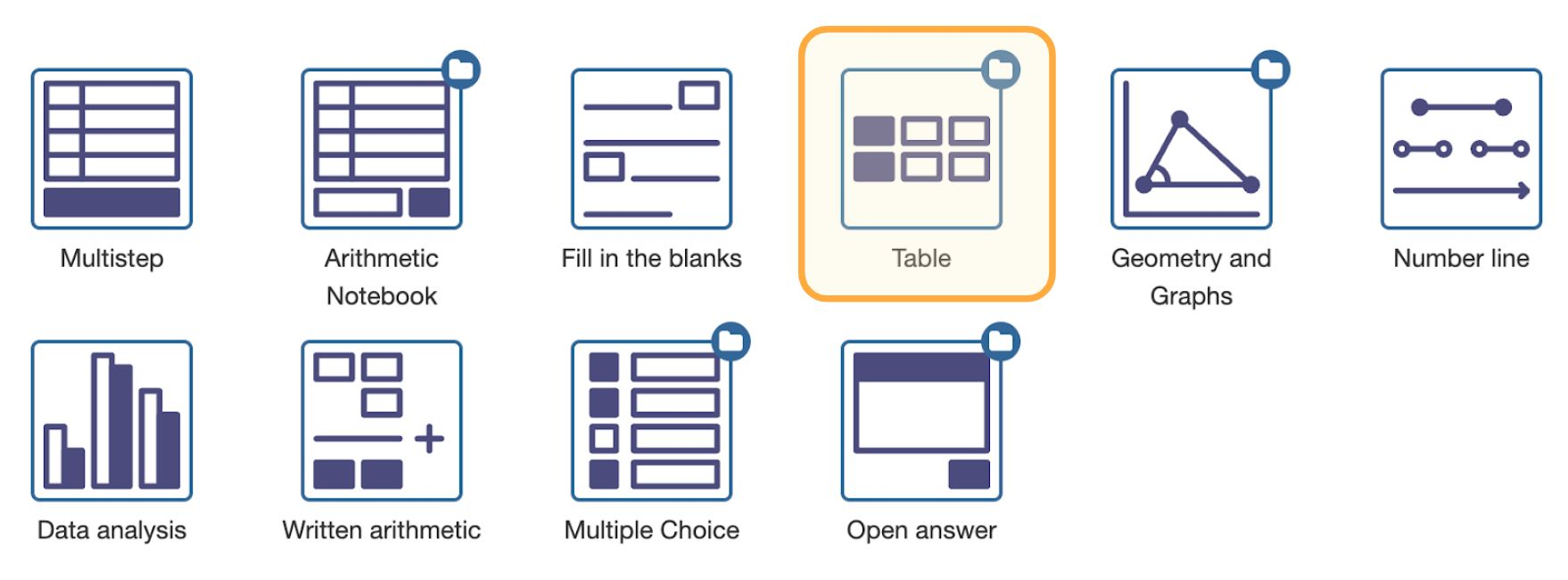# Math TableFigure: Selecting the Math Table question type.

Function tables, probability tables, sequences, statistics, and ratio tables are just a few examples of tables in mathematics. You can use the Math Table question type for all such use cases.

Tables rarely are just collections of numbers. Instead, the expressions in cells often share a common relation. You can configure such relations in Math Table to facilitate question creation and benefit from automatic hints and error feedback.